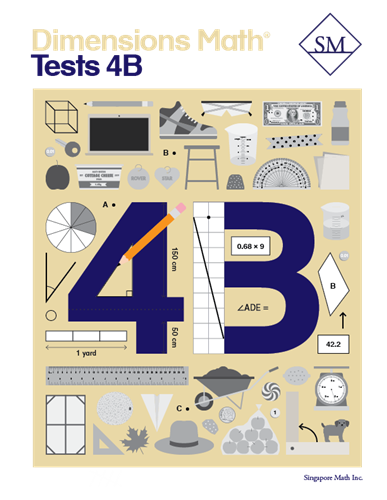# Dimensions Math Tests 4B

Chapter 10: Measurement
Test A
Test B
Chapter 11: Area and Perimeter
Test A
Test B
Chapter 12: Decimals
Test A
Test B
Chapter 13: Addition and Subtraction of Decimals
Test A
Test B
Continual Assessment 3
Test A
Test B
Chapter 14: Multiplication and Division of Decimals
Test A
Test B
Chapter 15: Angles
Test A
Test B
Chapter 16: Lines and Shapes
Test A
Test B
Chapter 17: Properties of Cuboids
Test A
Test B
Year-end Assessment
Test A
Test B

Dimensions Math® PreK-5 series features the progression, rigor, and pacing that define Singapore math. Throughout the series, five characters offer students suggestions on how to think about problems. They remind students of strategies they’ve learned and point out important information that encourages them to come up with their own solutions.

Textbook lessons begin with a task that allows students to apply their previous knowledge and learn through discussion. Once students have mastered a concept with the use of concrete and pictorial aids, they are ready to take on more abstract mathematical problem sets. They reach fluency by collecting various strategies along the way and applying them to new problems. Word problems give students a sense of math in real-world contexts.

Workbooks offer independent practice that follows a careful progression of exercise variation. Each textbook lesson includes a corresponding workbook exercise that starts with pictorial representation and progresses to more challenging abstract problems. Workbooks for PreK-2 are perforated.

Teacher’s Guides include lesson plans, mathematical background, games, helpful suggestions, and comprehensive resources for daily lessons. Lessons are laid out clearly and activities are designed for the whole class, small groups, and extension.

ISBN  9781947226548